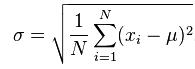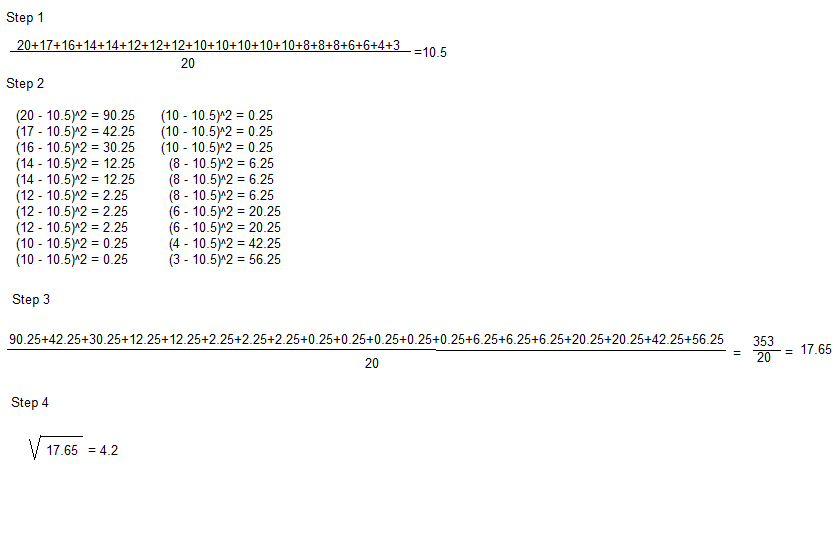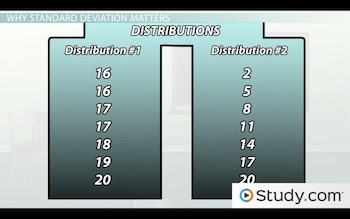# Using Standard Deviation and Bell Curves for Assessment

Lesson Transcript
Instructor: Jim Heald

Jim has taught undergraduate engineering courses and has a master's degree in mechanical engineering.

Standard deviation is often used by teachers to measure the difference in test scores from the average, while a bell curve represents a normal distribution in which most scores fall within the middle range. Learn about using standard deviation, bell curves, and how to use standard deviation for test score assessment. Updated: 08/20/2021

## Variability in Data

Imagine you're a teacher and you test your students on the United States Revolutionary War. How do you know what the scores mean in terms of how well the students did once you grade the test? You might be interested in how much variability, or difference, there was in the students' scores. In other words, did all of the students get similar scores to each other? Or did some students do really well, while other students in the same class did really poorly?

The purpose of this lesson is to talk about how you can learn about the variability of scores in a classroom environment and why that might matter. We're going to cover two important concepts: standard deviation of scores and how to interpret a normal distribution, also known as a bell curve.An error occurred trying to load this video.

Try refreshing the page, or contact customer support.

### You're on a roll. Keep up the good work!

Replay
Your next lesson will play in 10 seconds
• 0:05 Variability in Data
• 0:43 Standard Deviation
• 1:32 Calculating Standard Deviation
• 2:35 Why Standard Deviation Matters
• 4:46 Bell Curves
• 6:42 Lesson Summary
Save Save

Want to watch this again later?

Timeline
Autoplay
Autoplay
Speed Speed

## Standard Deviation

Let's start by using an example. Imagine you teach a class with 20 students, and they take a test with 20 multiple choice questions about the Revolutionary War. Imagine that the grades you get back from scoring their tests look like this:

 Student #1: 20 Student #11: 10 Student #2: 17 Student #12: 10 Student #3: 16 Student #13: 10 Student #4: 14 Student #14: 8 Student #5: 14 Student #15: 8 Student #6: 12 Student #16: 8 Student #7: 12 Student #17: 6 Student #8: 12 Student #18: 6 Student #9: 10 Student #19: 4 Student #10: 10 Student #20: 3

Now you want to know the basic variability within the classroom. So, did the students' scores kind of clump up all together, meaning the students all showed about the same amount of knowledge? Or did the scores vary widely from each other, meaning some students did great whereas other students failed the test?

The answer to this question can come very precisely from the standard deviation, which is a measurement that indicates how much a group of scores vary from the average.

So, let's look at our example from the Revolutionary War test.

## Calculating Standard DeviationThe standard deviation calculation looks complicated at first, but it's really quite simple if we take it step by step.1. We'll start by finding the mean, or average, of all the scores. To do this, we add up all the scores and divide by the total number of scores. This gives us a mean of 10.5.
2. The next step is to take each score, subtract the mean from it and square the difference. For example, looking at the top score of 20, we subtract 10.5 and then square the difference to get 90.25. We repeat this process for each score.
3. Now, we add up all our squared differences and divide by the total number of scores. This gives us 353/20, or 17.65.
4. The final step is to take the square root of this number, which is 4.2. This is the standard deviation of the scores.

## Why Standard Deviation Matters

Now that we have our standard deviation of 4.2, what the heck does that mean? Well, it just gives us an idea of how much the scores on the test clumped together. To understand this better, look at the two distributions of scores on the screen.The one on the left shows scores that are all very similar to each other. So, because the scores are all close together, the standard deviation is going to be very small. But, the one on the right shows scores that are all pretty different from each other (lots of high scores on the test, but also lots of failing grades on the test). For this distribution, we'd have a high number for our standard deviation.

So, why do we care about standard deviation at all? Well, a teacher would want to know this information because it might change how he or she teaches the material or how he or she constructs the test. Let's say that there's a small standard deviation because all of the scores clustered together right around the top, meaning almost all of the students got an A on the test. That would mean that the students all demonstrated mastery of the material. Or, it could mean that the test was just too easy! You could also get a small standard deviation if all of the scores clumped together on the other end, meaning most of the students failed the test. Again, this could be because the teacher did a bad job explaining the material or it could mean that the test is too difficult.

Most teachers want to get a relatively large standard deviation because it means that the scores on the test varied across the grade range. This would indicate that a few students did really well, a few students failed, and a lot of the students were somewhere in the middle. When you have a large standard deviation, it usually means that the students got all the different possible grades (like As, Bs, Cs, Ds, and Fs). So, the teacher can know that he or she taught the material correctly (because at least some of the students got an A) and the test was neither too difficult, nor too easy.

To unlock this lesson you must be a Study.com Member.

### Register to view this lesson

Are you a student or a teacher?